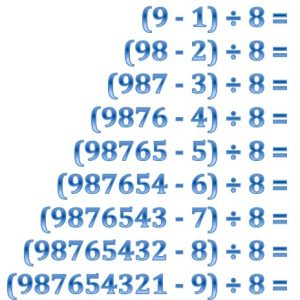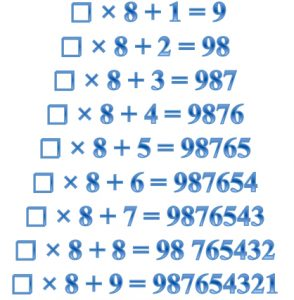Complete the calculations in the first list. Then use your answers to find the numbers to fill the boxes in the second list.

What do you notice about the connections between the equations: × 8 + 1 = 9 etc. and the calculations: (9 – 1) ÷ 8 = ? etc.

What do inverse operations have to do with the connections between these two lists?

You have been doing algebra!

The equations × 8 + 1 = 9 etc. can be written in the form   8 x + 1 = 9 etc.  where the letter x represents an unknown number and 8x means x multiplied by 8.

When you do algebra you are asked to solve equations. That means to find the number that the letter represents.

How would you use inverse operations to solve equations like   8 x + 1 = 9 etc.   ?